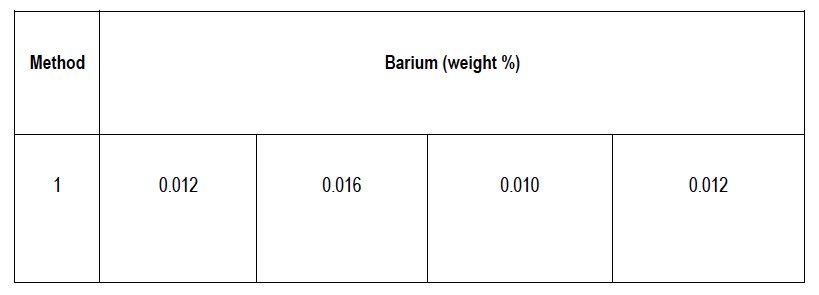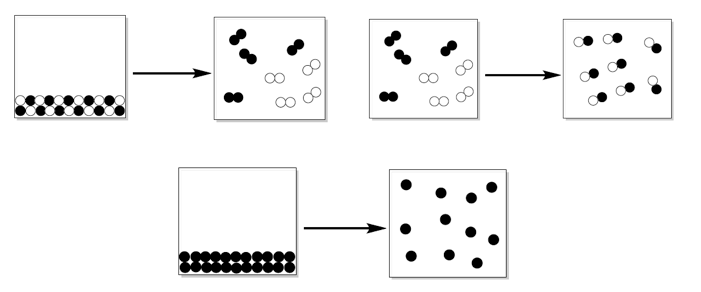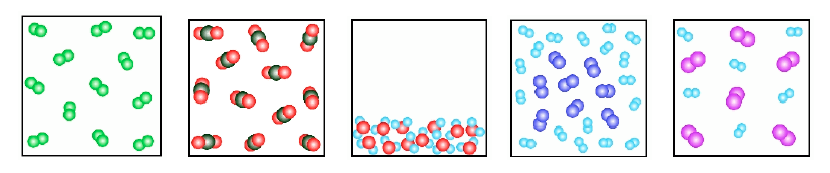Ch.1 - Intro to General ChemistryWorksheetSee all chapters
 Ch.1 - Intro to General Chemistry 2hrs & 53mins 0% complete Worksheet Ch.2 - Atoms & Elements 2hrs & 40mins 0% complete Worksheet Ch.3 - Chemical Reactions 3hrs & 25mins 0% complete Worksheet BONUS: Lab Techniques and Procedures 1hr & 38mins 0% complete Worksheet BONUS: Mathematical Operations and Functions 47mins 0% complete Worksheet Ch.4 - Chemical Quantities & Aqueous Reactions 3hrs & 30mins 0% complete Worksheet Ch.5 - Gases 3hrs & 47mins 0% complete Worksheet Ch.6 - Thermochemistry 2hrs & 28mins 0% complete Worksheet Ch.7 - Quantum Mechanics 2hrs & 35mins 0% complete Worksheet Ch.8 - Periodic Properties of the Elements 1hr & 57mins 0% complete Worksheet Ch.9 - Bonding & Molecular Structure 2hrs & 5mins 0% complete Worksheet Ch.10 - Molecular Shapes & Valence Bond Theory 1hr & 31mins 0% complete Worksheet Ch.11 - Liquids, Solids & Intermolecular Forces 3hrs & 40mins 0% complete Worksheet Ch.12 - Solutions 2hrs & 17mins 0% complete Worksheet Ch.13 - Chemical Kinetics 2hrs & 22mins 0% complete Worksheet Ch.14 - Chemical Equilibrium 2hrs & 26mins 0% complete Worksheet Ch.15 - Acid and Base Equilibrium 4hrs & 42mins 0% complete Worksheet Ch.16 - Aqueous Equilibrium 3hrs & 48mins 0% complete Worksheet Ch. 17 - Chemical Thermodynamics 1hr & 44mins 0% complete Worksheet Ch.18 - Electrochemistry 2hrs & 58mins 0% complete Worksheet Ch.19 - Nuclear Chemistry 1hr & 33mins 0% complete Worksheet Ch.20 - Organic Chemistry 3hrs 0% complete Worksheet Ch.22 - Chemistry of the Nonmetals 2hrs & 1min 0% complete Worksheet Ch.23 - Transition Metals and Coordination Compounds 1hr & 54mins 0% complete Worksheet

# Intro to Chem Additional Problems

See all sections

Test your skills on the following problems.

###### Standard Deviation

Practice: The barium content of a metal ore was analyzed several times by a percent composition process.

a) Calculate the mean, median and mode.

b) Calculate the standard deviation.###### Chemical Composition

Practice: Determine which of the following represents a physical change and a chemical change.Practice: Answer each of the following questions based on the images provided below.

a) Which of the following images represents an elemental gas?

b) Which of the following images represents a heterogeneous mixture?

c) Which of the following images represents a liquid?Practice: Which of the following represents a physical property?

a) Mercury is a liquid at room temperature.

b) Alkanes burn spontaneously.

c) CO2 (s) -----> CO2 (g)

d) 2 H2 (g) + O2 (g) ----->  2 H2O (g)

e) The rusting of a car.

Practice: A cigarette lighter contains the substance butane, C4H10, with the given properties.

a) The lighter contains 10 g of butane

b) the density of butane is 0.57 g/cm3

c) The freezing point of the butane is -138oC

d) Butane is combustible in air.

i) Which of the following features is a chemical change?

ii) Which of the following properties are intensive properties?

Practice: Which of the following properties about an unknown metal represent chemical properties?

I. It has a bluish-green color.

II. Upon exposure to air the metal experiences corrosion.

III. It has a density of 4.36 g/cm3.

IV. It has a boiling point of 522oC.

V. It conducts electricity.

###### Density

Practice: An empty flask has a volume of 250 mL. When water is placed inside the flask (d = 0.9983 g/mL) the volume is 124.3 mL. What is the mass (in grams) of the water inside the flask?

Practice: If a statue at an art gallery is covered in 279 kg of copper. If the copper on the statue has a thickness of 0.0055 cm, what surface area is covered (in square meters)? Copper has a density of 8.96 g/cm3.

###### Dimensional Analysis

Practice: A Volkswagen diesel engine consumes diesel at a rate of 20.17 L per hour. If the density of the diesel is 0.850 g/mL. What is the mass (in mg) of diesel needed to drive for a continuous 4.3 hours?

Practice: If the charge and mass of one proton is 1.60218 x 10-19 C and 1.673 x 10-30 g respectively, what is the charge of 378 kg of protons.

Practice: A cylindrical tube has a length that is 15.2 cm and is filled with bromine liquid. If the mass of the bromine is 112.3 g and has a density of 25.3 g/mL. What is the inner diameter of the glass tube? ( V = π • r2 • h )

Practice: A large body of water contaminated with lead has an average depth of 750 m, a total area of 1.25 x 105 km2, and an average of 5.8 x 10-12 g/L of dissolved lead. How many milligrams of lead are in this large body of water?

Practice: Calculate the mass (in grams) of a golden sphere with a diameter of 30.0 mm. The density of gold is 19.3 g/cm3. (Vsphere = 4/3 π r3).